Ch 6. Structures Multimedia Engineering Statics 2-D Trusses: Joints 2-D Trusses: Sections 3-D Trusses Frames and Machines
 Chapter 1. Basics 2. Vectors 3. Forces 4. Moments 5. Rigid Bodies 6. Structures 7. Centroids/Inertia 8. Internal Loads 9. Friction 10. Work & Energy Appendix Basic Math Units Sections Search eBooks Dynamics Fluids Math Mechanics Statics Thermodynamics Author(s): Kurt Gramoll ©Kurt GramollSTATICS - CASE STUDY SOLUTION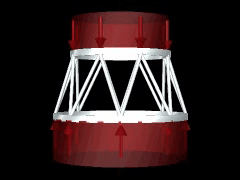Loading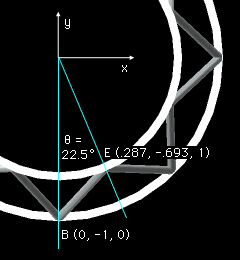Top View at Point B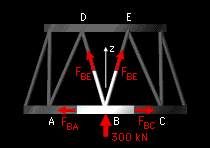FBD of Joint B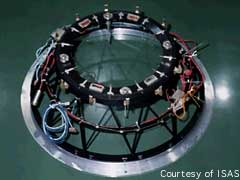Example of Space Truss Since all members are symmetrical around the inter-stage structure, all members will have the same load. Using the method of joints, the forces at joint B on the bottom ring is analyzed by summing the forces,      ΣFB = 0 The free-body diagram includes the external thrust load and all member forces acting at joint B. Since Joint B is in static equilibrium, the summation of all force vectors must equal zero. Since this is a 3D problem, all forces will be represented in the vector i, j, k format. The total thrust load of 2,400 kN is evenly distributed around the inter-stage structure so each joint will have to withstand a vertical load of 300 kN.      FT = 300 k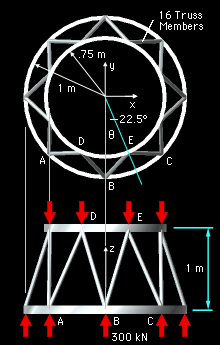Truss Diagram Use the location of points B and E to define the unit directional vector of FBE.      Ex = 0.75 sin22.5 = 0.2870 m      Ey = -0.75 cos22.5 = -0.6929 m      Ez = 1.0 m      Bx = 0 m      By = -1 m      Bz = 0 m The length of member BE is      BE = ((0.2870 - 0)2 + (-0.6929 - (-1))2                             + (1 - 0)2 )0.5           = 1.085 m The vector FBE in i, j, k format is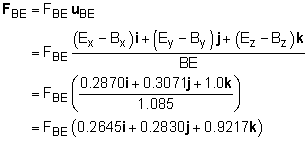The member force FBD is similar to FBE except the x component is reversed. The magnitudes will be the same:      FBD = FBE (-0.2645i + 0.2830j + 0.9217k) If forces are summed in the z direction, ΣFz = 0, only one unknown remains, FBE. Solving for FBE gives      2 FBE 0.9217 + 300 = 0      FBE = -162.7 kN   compression

Practice Homework and Test problems now available in the 'Eng Statics' mobile app
Includes over 500 problems with complete detailed solutions.
Available now at the Google Play Store and Apple App Store.